# INTERPRET LINEAR GRAPHS WRITE LINEAR EQUATIONS SECTION 5

• Slides: 15INTERPRET LINEAR GRAPHS & WRITE LINEAR EQUATIONS SECTION 5. 4 C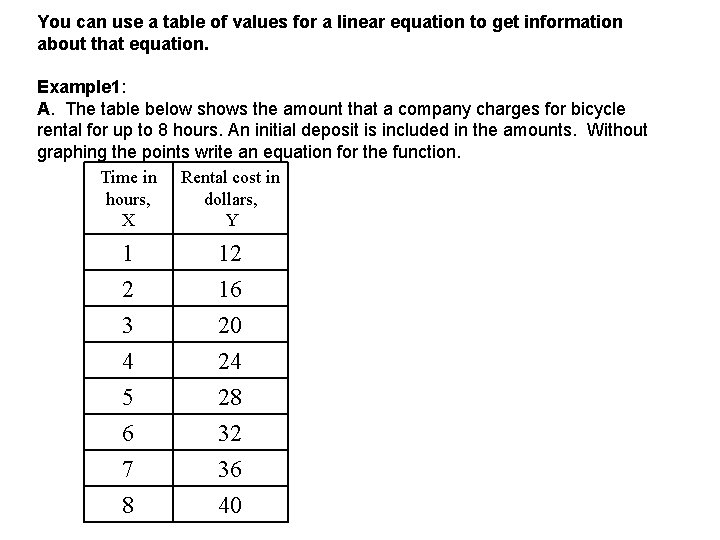You can use a table of values for a linear equation to get information about that equation. Example 1: A. The table below shows the amount that a company charges for bicycle rental for up to 8 hours. An initial deposit is included in the amounts. Without graphing the points write an equation for the function. Time in Rental cost in hours, dollars, X Y 1 12 2 3 4 5 6 7 8 16 20 24 28 32 36 40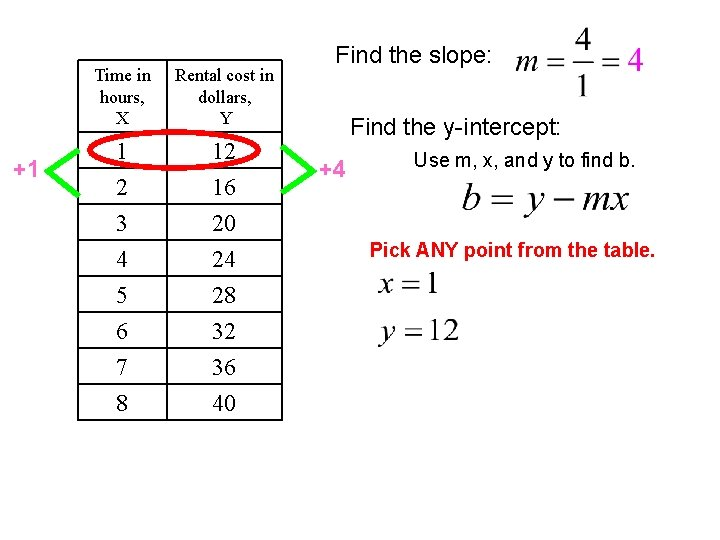+1 Time in hours, X Rental cost in dollars, Y 1 12 2 3 4 5 6 7 8 16 20 24 28 32 36 40 Find the slope: Find the y-intercept: +4 Use m, x, and y to find b. Pick ANY point from the table.+1 Time in hours, X Rental cost in dollars, Y 1 12 2 3 4 5 6 7 8 16 20 24 28 32 36 40 Find the slope: Find the y-intercept: +4 Use m, x, and y to find b. Pick ANY point from the table.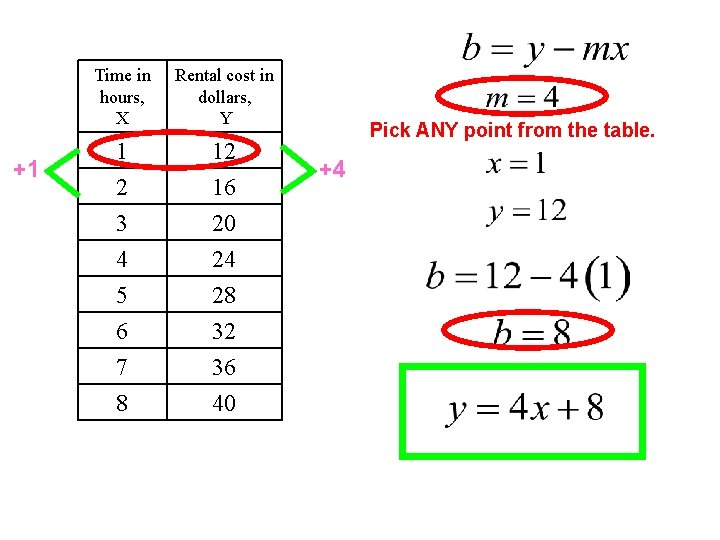+1 Time in hours, X Rental cost in dollars, Y 1 12 2 3 4 5 6 7 8 16 20 24 28 32 36 40 Pick ANY point from the table. +4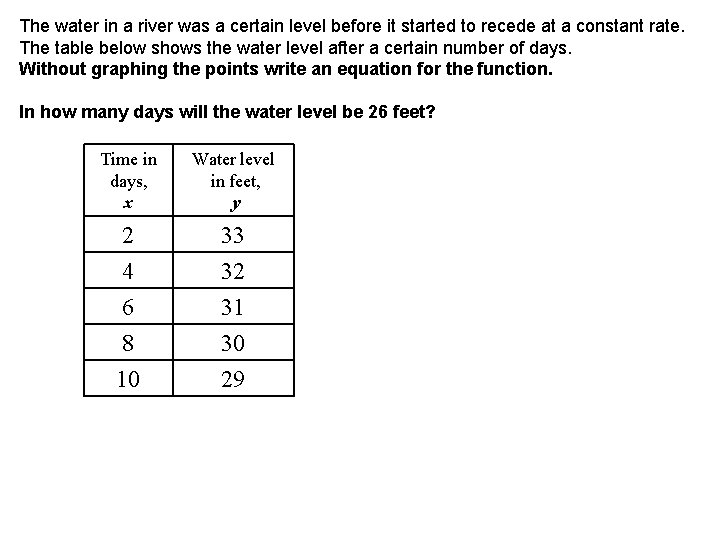The water in a river was a certain level before it started to recede at a constant rate. The table below shows the water level after a certain number of days. Without graphing the points write an equation for the function. In how many days will the water level be 26 feet? Time in days, x Water level in feet, y 2 33 4 6 8 10 32 31 30 29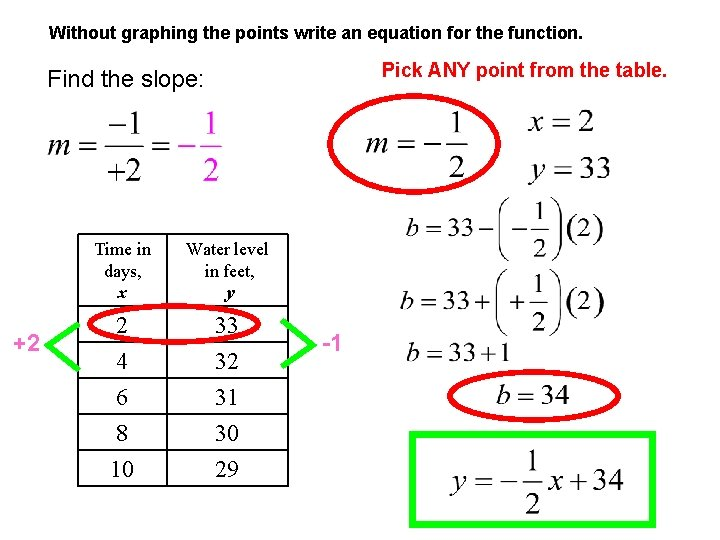Without graphing the points write an equation for the function. Pick ANY point from the table. Find the slope: +2 Time in days, x Water level in feet, y 2 33 4 6 8 10 32 31 30 29 -1The water in a river was a certain level before it started to recede at a constant rate. The table below shows the water level after a certain number of days. Without graphing the points write an equation for the function. In how many days will the water level be 26 feet? Time in days, x Water level in feet, y 2 33 4 6 8 10 32 31 30 29 16 If y = 26 feet, what is x? s y da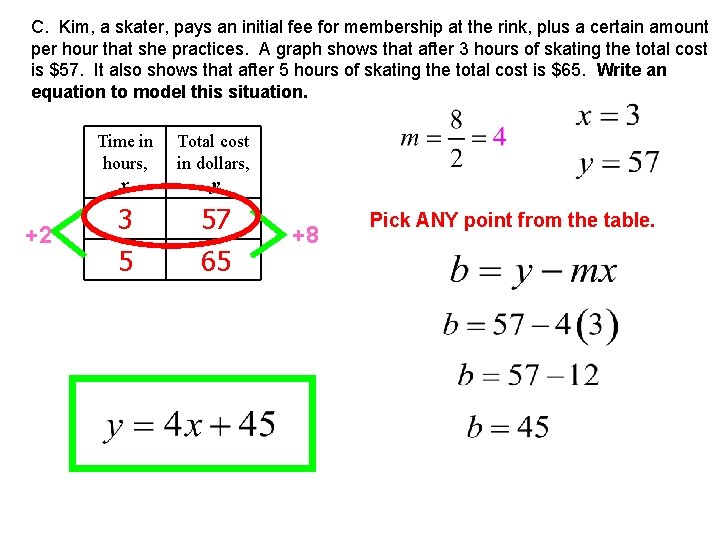C. Kim, a skater, pays an initial fee for membership at the rink, plus a certain amount per hour that she practices. A graph shows that after 3 hours of skating the total cost is \$57. It also shows that after 5 hours of skating the total cost is \$65. Write an equation to model this situation. +2 Time in hours, x Total cost in dollars, y 3 5 57 65 +8 Pick ANY point from the table.Quite often you will be given two points on a line and asked to write the linear equation. 1. Find the slope. 2. Substitute the values for the slope and x and y. 3. Find the value of the y-intercept. 4. Write your equation in slope intercept form.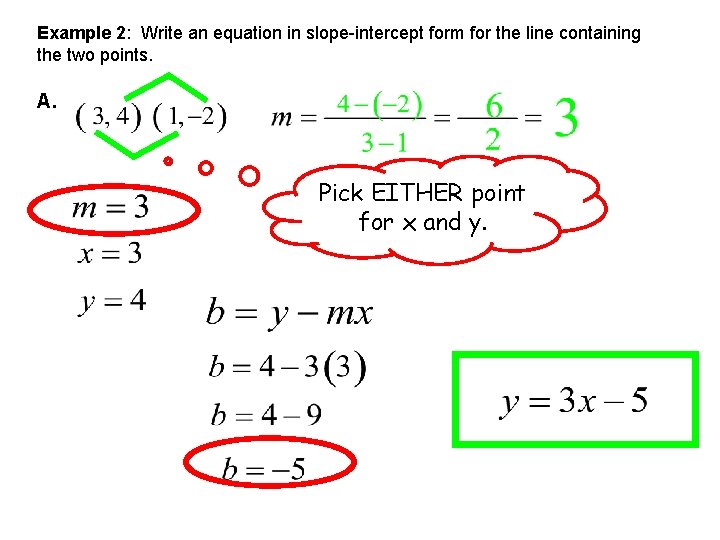Example 2: Write an equation in slope-intercept form for the line containing the two points. A. Pick EITHER point for x and y.Example 2: Write an equation in slope-intercept form for the line containing the two points. B. Pick EITHER point for x and y.Example 2: Write an equation in slope-intercept form for the line containing the two points. C. Pick EITHER point for x and y.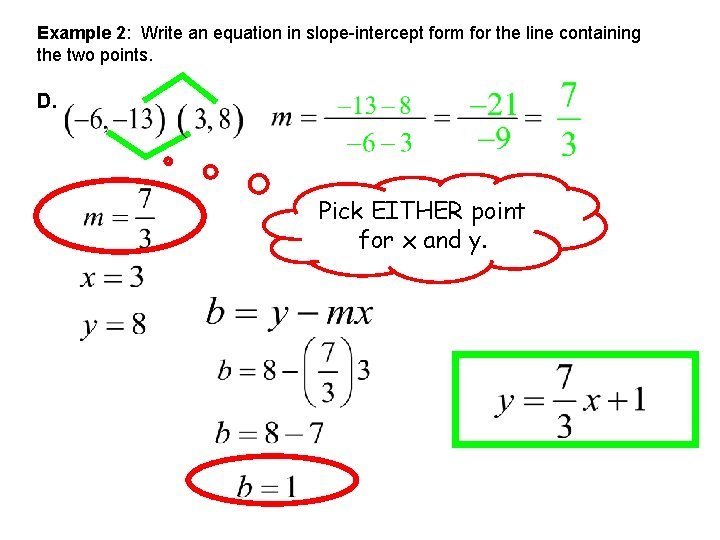Example 2: Write an equation in slope-intercept form for the line containing the two points. D. Pick EITHER point for x and y.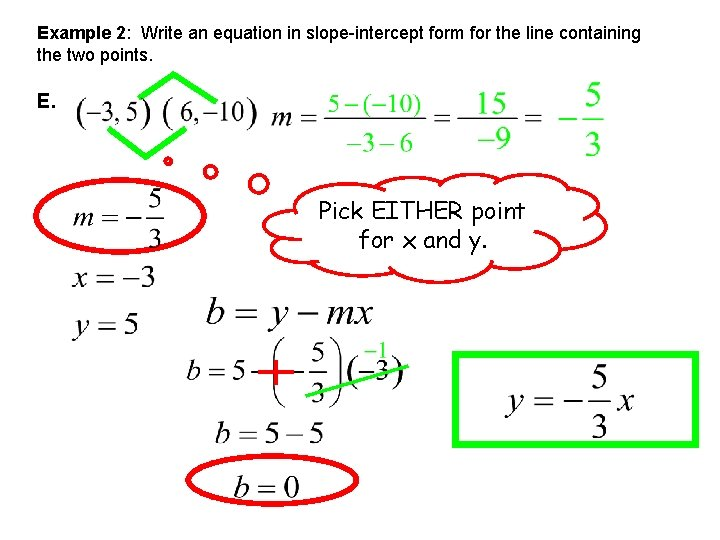Example 2: Write an equation in slope-intercept form for the line containing the two points. E. Pick EITHER point for x and y.